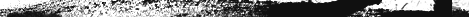G A T E F O R S T U D E N T S - C-aptitude (41-60)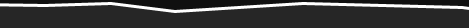41)       #include<stdio.h>
main()
{
struct xx
{
int x=3;
char name[]="hello";
};
struct xx *s=malloc(sizeof(struct xx));
printf("%d",s->x);
printf("%s",s->name);
}
Compiler Error
Explanation:
Initialization should not be done for structure members inside the structure declaration

42)       #include<stdio.h>
main()
{
struct xx
{
int x;
struct yy
{
char s;
struct xx *p;
};
struct yy *q;
};
}
Compiler Error
Explanation:
in the end of nested structure yy a member have to be declared.

43)       main()
{
extern int i;
i=20;
printf("%d",sizeof(i));
}
Explanation:
extern declaration specifies that the variable i is defined somewhere else. The compiler passes the external variable to be resolved by the linker. So compiler doesn't find an error. During linking the linker searches for the definition of i. Since it is not found the linker flags an error.

44)       main()
{
printf("%d", out);
}
int out=100;
Compiler error: undefined symbol out in function main.
Explanation:
The rule is that a variable is available for use from the point of declaration. Even though a is a global variable, it is not available for main. Hence an error.

45)       main()
{
extern out;
printf("%d", out);
}
int out=100;
100
Explanation:
This is the correct way of writing the previous program.

46)       main()
{
show();
}
void show()
{
printf("I'm the greatest");
}
Compier error: Type mismatch in redeclaration of show.
Explanation:
When the compiler sees the function show it doesn't know anything about it. So the default return type (ie, int) is assumed. But when compiler sees the actual definition of show mismatch occurs since it is declared as void. Hence the error.
The solutions are as follows:
1. declare void show() in main() .
2. define show() before main().
3. declare extern void show() before the use of show().

47)       main( )
{
int a = {{{2,4},{7,8},{3,4}},{{2,2},{2,3},{3,4}}};
printf(“%u %u %u %d n”,a,*a,**a,***a);
printf(“%u %u %u %d n”,a+1,*a+1,**a+1,***a+1);
}
100, 100, 100, 2
114, 104, 102, 3
Explanation:
The given array is a 3-D one. It can also be viewed as a 1-D array.

 2 4 7 8 3 4 2 2 2 3 3 4
100 102 104 106 108   110 112 114 116   118   120   122

thus, for the first printf statement a, *a, **a give address of first element . since the indirection ***a gives the value. Hence, the first line of the output.
for the second printf a+1 increases in the third dimension thus points to value at 114, *a+1 increments in second dimension thus points to 104, **a +1 increments the first dimension thus points to 102 and ***a+1 first gets the value at first location and then increments it by 1. Hence, the output.

48)       main( )
{
int a[ ] = {10,20,30,40,50},j,*p;
for(j=0; j<5; j++)
{
printf(“%d” ,*a);
a++;
}
p = a;
for(j=0; j<5; j++)
{
printf(“%d ” ,*p);
p++;
}
}
Compiler error: lvalue required.

Explanation:
Error is in line with statement a++. The operand must be an lvalue and may be of any of scalar type for the any operator, array name only when subscripted is an lvalue. Simply array name is a non-modifiable lvalue.

49)       main( )
{
static int a[ ]   = {0,1,2,3,4};
int *p[ ] = {a,a+1,a+2,a+3,a+4};
int **ptr = p;
ptr++;
printf(“n %d %d %d”, ptr-p, *ptr-a, **ptr);
*ptr++;
printf(“n %d %d %d”, ptr-p, *ptr-a, **ptr);
*++ptr;
printf(“n %d %d %d”, ptr-p, *ptr-a, **ptr);
++*ptr;
printf(“n %d %d %d”, ptr-p, *ptr-a, **ptr);
}
111
222
333
344
Explanation:
Let us consider the array and the two pointers with some address
a
 0 1 2 3 4
100      102      104      106      108
p
 100 102 104 106 108
1000    1002    1004    1006    1008
ptr
 1000
2000
After execution of the instruction ptr++ value in ptr becomes 1002, if scaling factor for integer is 2 bytes. Now ptr – p is value in ptr – starting location of array p, (1002 – 1000) / (scaling factor) = 1, *ptr – a = value at address pointed by ptr – starting value of array a, 1002 has a value 102 so the value is (102 – 100)/(scaling factor) = 1, **ptr is the value stored in the location pointed by the pointer of ptr = value pointed by value pointed by 1002 = value pointed by 102 = 1. Hence the output of the firs printf is 1, 1, 1.
After execution of *ptr++ increments value of the value in ptr by scaling factor, so it becomes1004. Hence, the outputs for the second printf are ptr – p = 2, *ptr – a = 2, **ptr = 2.
After execution of *++ptr increments value of the value in ptr by scaling factor, so it becomes1004. Hence, the outputs for the third printf are ptr – p = 3, *ptr – a = 3, **ptr = 3.
After execution of ++*ptr value in ptr remains the same, the value pointed by the value is incremented by the scaling factor. So the value in array p at location 1006 changes from 106 10 108,. Hence, the outputs for the fourth printf are ptr – p = 1006 – 1000 = 3, *ptr – a = 108 – 100 = 4, **ptr = 4.

50)       main( )
{
char *q;
int j;
for (j=0; j<3; j++) scanf(“%s” ,(q+j));
for (j=0; j<3; j++) printf(“%c” ,*(q+j));
for (j=0; j<3; j++) printf(“%s” ,(q+j));
}
Explanation:
Here we have only one pointer to type char and since we take input in the same pointer thus we keep writing over in the same location, each time shifting the pointer value by 1. Suppose the inputs are MOUSE, TRACK and VIRTUAL. Then for the first input suppose the pointer starts at location 100 then the input one is stored as
 M O U S E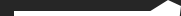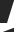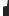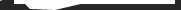Site Maintained by Saravanan.R.R. NithinRaj.PFor Job Searching www.naukri.com www.freshersworld.com www.jobsearch.monsterindia.com www.recruit.net www.timesjobs.com www.jobsearchworld.com www.placementindia.com www.bestjobsindia.in www.jobsahead.com www.jobsearch.co.in www.jobsearchinformation.com www.jobconsultancy.com www.marketingcrossing.com www.mobile.monsterindia.com www.hinduonnet.comTutorials Searching www.howstuffworks.com www.tutor.com www.en.wikipedia.org www.mathguru.com www.knowledgeonlineservices.com www.mygretutor.comEnglish Tutor Searching www.englishgrammartutor.com www.theenglishtutor.com www.learnenglish.de www.tolearnenglish.com www.englishclub.com www.english-at-home.com www.learn-english.co.il www.learnenglish.beFor Entrance Exam Details Searching www.winentrance.com www.entranceexamindia.com www.studyplaces.com www.entranceexam.info www.indiastudychannel.com www.edudiary.com www.successcds.net